Courses

# Competition Level Test: Indefinite Integration- 1

## 30 Questions MCQ Test Mathematics For JEE | Competition Level Test: Indefinite Integration- 1

Description
This mock test of Competition Level Test: Indefinite Integration- 1 for JEE helps you for every JEE entrance exam. This contains 30 Multiple Choice Questions for JEE Competition Level Test: Indefinite Integration- 1 (mcq) to study with solutions a complete question bank. The solved questions answers in this Competition Level Test: Indefinite Integration- 1 quiz give you a good mix of easy questions and tough questions. JEE students definitely take this Competition Level Test: Indefinite Integration- 1 exercise for a better result in the exam. You can find other Competition Level Test: Indefinite Integration- 1 extra questions, long questions & short questions for JEE on EduRev as well by searching above.
QUESTION: 1

### A spherical iron ball 10 cm in radius is coated with a layer of ice of uniform thickness that melts at a rate of 50 cm³/min. When the thickness of ice is 15 cm, then the rate at which the thickness of ice decreases, is

Solution: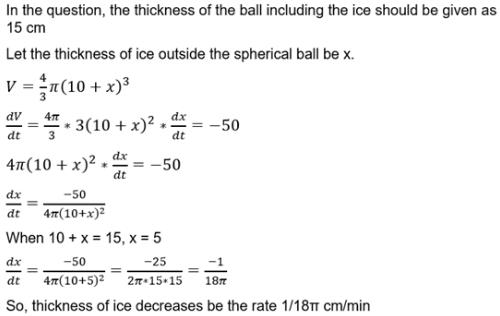QUESTION: 2

### Area of the greatest rectangle that can be inscribed in the ellipse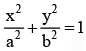is

Solution:

Let a point on the ellipse be P (a cosθ, b sinθ)
So, In the rectangle formed as this point as one of the vertices,
(Length)/2 = a cosθ
(Width)/2 = b sinθ
Area of the rectangle = (2a cosθ)(2b sinθ)
= 4ab(sinθ)(cosθ)
= 2ab sin(2θ)
Max (Area of the rectangle) = 2ab

QUESTION: 3

### Let f(x) be differentiable for all x. If f (1) =-2 and f '(x)³ ≥2 x ∈ (1/6)  then

Solution:

By LMVT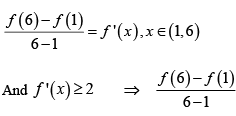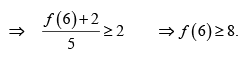QUESTION: 4

The normal to the curve x = a ( cos θ + θ sin θ) , y = a (sin θ - θ cos θ) at any point ' θ' is such that

Solution: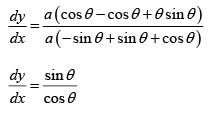Equation of normal is
(y -θ sinq- cosθ )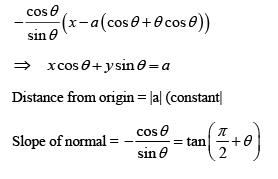QUESTION: 5

The function f (x) = x/2+2/x has a local minimum at

Solution: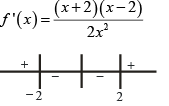Signs of f ' (x )

f ' (x ) changes sign as x crosses 2.

f (x ) has minima at x = 2.

QUESTION: 6

A value of c for which the conclusion of Mean value Theorem holds for the function f ( x ) = loge x on the interval [1, 3] is

Solution: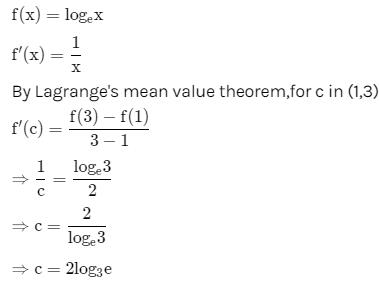QUESTION: 7

The function f ( x ) = tan-1 (sin x cos x)  is an increasing function in

Solution: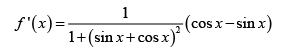QUESTION: 8

Suppose the cubic x3 - px + q = 0 has three distinct real roots where p > 0 and q > 0. Then, which one of the following holds?

Solution:

Graph of  y = x2 - px + q cuts x-axis at three distinct pints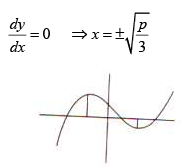Maximum at  x = -√p/3 , minima at  x = √p/3

QUESTION: 9

The shortest distance between the line y - x= 1 and the curve x =y2 is

Solution:

Condition for shortest distance is slope of tangent to x = y² must be same as slope of line y = x + 1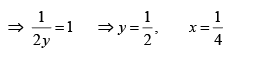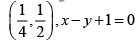shortest distance =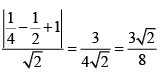QUESTION: 10

Given p ( x ) = x 4 + ax3 + bx 2 + cx + d such that x =0 is the only real root of p ' ( x ) = 0 .

If p ( -1) < p (1) , then in the interval [-1,1]

Solution: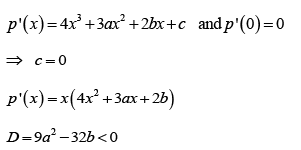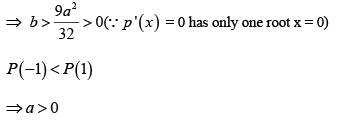p ' (x ) has only one change of sign.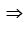x = 0 is a point of minima.

P(-1) =1- a + b + d P(0) = d

P(1) =1+ a + b + dP ( -1) < P (1) , P ( 0) < P (1) , P ( -1) > P ( 0)P (-1) is not minimum but P(1) is maximum

QUESTION: 11

The equation of the tangent to the curve y= x+4/x2 that is parallel to the x-axis is

Solution: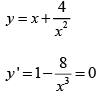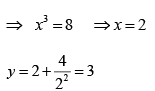(2, 3) is point of contact thus y = 3 is tangent

QUESTION: 12

Let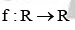be defined by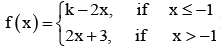If f has a local minimum at x = - 1, then a possible value of k is

Solution: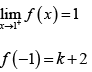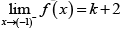∴f has a local minimum at x = - 1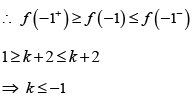Possible value of k is – 1

QUESTION: 13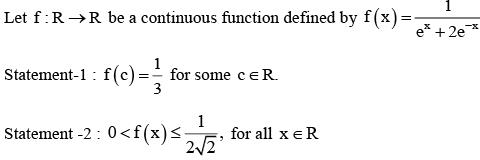Solution: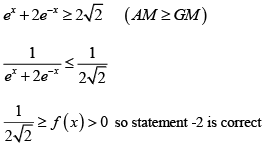As f(x) is continuous and 1/3 belongs to range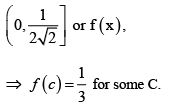QUESTION: 14

The normal to the curve  x2 + 2xy - 3y2 = 0 at 1,1

Solution: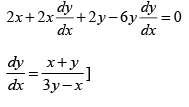slope of normal at (1, 1) = - 1

∴ Equation of normal y -1 = -1(x -1)

y +x= 2
Solving we get point as (1, 1) and (1/-1/3)

QUESTION: 15

Consider the function, f ( x ) =| x - 2 | + | x - 5 | .x∈ R

Statement -1 : f ' ( 4) = 0

Statement -2 : f is continous in [2, 5], differentiable in (2, 5) and f ( 2) = f ( 5)

Solution:

f (x ) is constant is [2, 5]

QUESTION: 16

A spherical balloon is filled with 4500 π cubic meters of helium gas. If a leak in the balloon causes the gas to escape at the rate of 75 π cubic meters per minute, then the rate (in metres per minute) at which the radius of the balloon decreases 49 minutes after the leakage began is

Solution: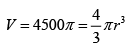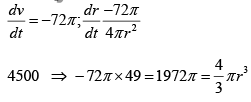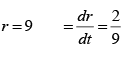QUESTION: 17

Let a, b ∈ R be such that the function f given by f ( x ) = In | x | +bx 2 + ax, x ≠ 0 has extreme values at x =-1 and x = 2.

Statement – 1: f has local maximum at x = - 1 and at x = 2.

Statement-2 : a=1/2 and =-1/4

Solution: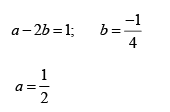QUESTION: 18

The real number k for which the equation, 2x3 + 3x + k = 0 has two distinct real roots in [0, 1]

Solution:

f ' ( x ) = 6 x2+ 3
⇒ so f(x) is increasing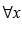equation cannot have 2 distinct roots.

QUESTION: 19

The function f ( x ) = sin4 x+ cos4 x is increasing if

Solution: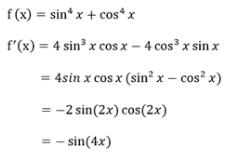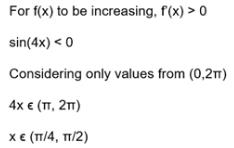QUESTION: 20

​Let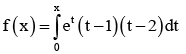dt then f decreasing in the interval

Solution: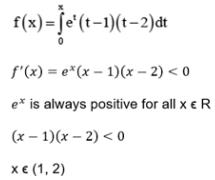QUESTION: 21

The two curves x3 - 3xy2 + 2 = 0 and 3x 2 y - y3 - 2 = 0

Solution: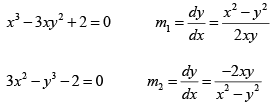mm = -1 ⇒ Curves are orthogonal

QUESTION: 22

The greatest value of f (x) = (x + 1)1/3 - (x - 1)1/3 on [0, 1] is

Solution: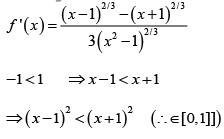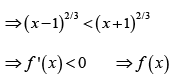is decreasing on [0, 1].
Greatest value of f (x ) is f ( 0) = 1 - ( -1) = 2

QUESTION: 23

The function f ( x ) = cot -1 x+ x increases in the interval

Solution: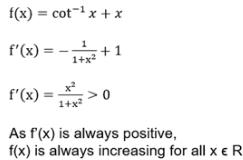QUESTION: 24

The real number x (x > 0) when added to its reciprocal gives the minimum sum at x equals

Solution: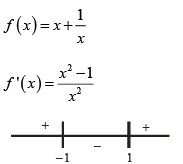Signs of f ' (x )

Minimum occurs at x = 1

QUESTION: 25

If the function f ( x ) = 2x3 - 9ax2 + 12a 2x + 1, where a >0 , attains its maximum and minimum at p and q respectively such that p² = q, then 'a' equals

Solution: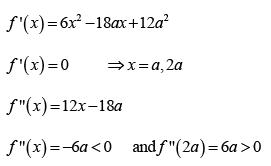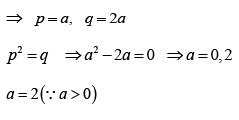QUESTION: 26

If 2a + 3b + 6c = 0, then at least one root of the equation ax 2 + bx + c = 0 lies in the interval

Solution: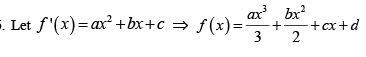f ( 0) = df (1) (∵ 2a + 3b + 6x = 0)

By Rolls theorem, at least one root of f ' (x ) =0 lies in (0, 1)

QUESTION: 27

A point on the parabola y2 = 18x at which the ordinate increases at twice the rate of the abscissa, is

Solution: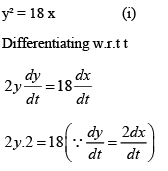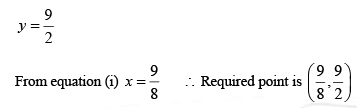QUESTION: 28

The normal to the curve  x = a (1 + cos θ) , y = a sin θ at 'θ ' always passes through the fixed point

Solution:

x = a (1 + cosθ ) , ya = sinθ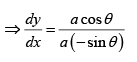Equation of normal at point ( a (1 + cosθ ) ,a sinθ ) is
y - a sinθ = sinθ/cosθ (x-a(1+cosθ))
y cosθ = x- a ) sinθ

It is clear that normal passes through fixed point (a, 0)

QUESTION: 29

Angle between the tangents to the curve y = x 2 - 5x + 6 at the points (2, 0) and (3, 0) is

Solution: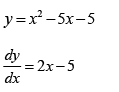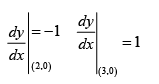Product of slopes = -1 ⇒ angle between tangents is π/2

QUESTION: 30

A function is matched below against an interval where it is supposed to be increasing, Which of the following pairs is incorrectly matched?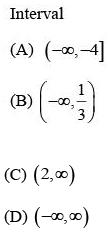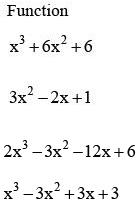Solution:

Let  f (x) = x2 + 6x +6 ⇒ f (x) = 3x (x +4)

Increasing in (-∞,-4) Let ( ) 2 2
f2 (x) = 3x2 - 2x + 1

Track your progress, build streaks, highlight & save important lessons and more!

### Similar Content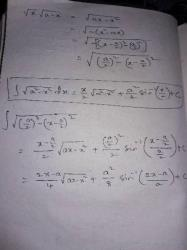### Related tests